# Using Algorithms to Solve Math Problems

Instructor: Michael Quist

Michael has taught college-level mathematics and sociology; high school math, history, science, and speech/drama; and has a doctorate in education.

Algorithms are precise sets of instructions that tell us exactly how to do something. In this lesson, we will explore how to use algorithms to solve math problems.

## What are Algorithms?

You're staring blankly at two numbers that you're supposed to divide, and you just can't think of what to do first. If only you had a set of instructions!

An algorithm is a set of steps that, if you do it right, will always take you to the goal. It is like a set of instructions for building a model plane, or a recipe for making a cake. In mathematics, an algorithm will always point you toward the right answer.

## Using an Algorithm to Add a Column of Numbers

A good example of using an algorithm for math is a 'left to right' approach for adding a column of numbers.

Say you start with an ugly group of numbers that you have to add together by hand (no calculator, PC, smart phone, etc.--you know, Stone Age stuff!)

You have 5280, 495, and 1520, and you want the total. So, you grab a piece of paper (or write on the cloth napkin, but don't tell anyone!), and you think about the algorithm.

Here's the algorithm:

1. Put the numbers in a column, matching up the number places (you know, the 1's go with the 1's, the 10's with the 10's, etc.).
2. Draw a line under them, to separate the numbers you're adding from your results.
3. Add the 1000's column and write the total under the line in the 1000's column.
4. Add the 100's column and write the total in the 100's column beneath your 1000's total.
5. Add the 10's column and write that total in the 10's column beneath your 100's total.
6. Add the 1's column and write that total in the 1's column beneath your 10's total.
7. Adjust the 1's column: If the total of the 1's is more than 9, then you have one or more 10's in your 1's column. They have to be relocated to the 10's column. Write the number of 10's in the 10's column, and leave what's left in the 1's column.
8. Adjust the 10's column: If you have any 100's in the 10's column, remove them from the 10's column and place that number of 100's in the 100's column.
9. Adjust the 100's column: If there are any 1000's in the 100's column, relocate them to the 1000's column.
10. Total up each of your column results, and write them next to each other in a row. You should now have the total (sum) of your original numbers.

Let's put these steps into action to solve our problem.

First, we'll put the numbers in a column for addition.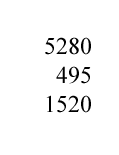Then, draw a line underneath to set apart your results.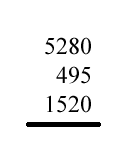Looking at the 1000's column (the one on the far left), we can see that only two of the numbers actually have a digit for 1000's, so we'll add those together (5000 + 1000 = 6000). You can write in the 0's for the 1000's if you want to, but we'll just keep the number of 1000's in the 1000's column to show that the number represents 1000's.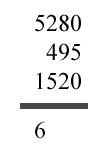Looking at the 100's column, we can see that there are two 100's in the first number, four 100's in the second one, and five 100's in the third number. Adding the 100's together, we get 11 of them, which we'll write in the 100's column under our 1000's total. Notice that we do have one 1000 in our 1100, but we'll deal with him later.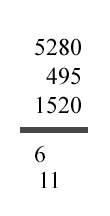Next we'll add the 10's. We get 19 of them, or 190. We notice a 100 in that total, and we'll have to move him to the 100's column later, but let's check the 1's first.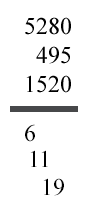Adding the 1's together (two 0's and a 5), we get a total of 5 for the 1's column. Now we're ready to start wrapping this up.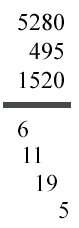We'll pull the 5 down to the bottom. No 10's to carry over to the next column.

Moving over to our nineteen 10's we'll move one 100 to the next column, and bring the 9 down to the bottom.

To unlock this lesson you must be a Study.com Member.

### Register to view this lesson

Are you a student or a teacher?

### Unlock Your Education

#### See for yourself why 30 million people use Study.com

##### Become a Study.com member and start learning now.
Back
What teachers are saying about Study.com

### Earning College Credit

Did you know… We have over 160 college courses that prepare you to earn credit by exam that is accepted by over 1,500 colleges and universities. You can test out of the first two years of college and save thousands off your degree. Anyone can earn credit-by-exam regardless of age or education level.